## Ultimate Guide To What Is a Watthour

Have you ever wondered how much energy your laptop requires and how much power it consumes? This may look like we are asking the same question in two different ways, but that's not true. When determining the electrical power consumption of an appliance, you will come across two different quantities - watts and watthours.

But what is a watt, and what is a watthour? How do they differ? While watts measure the power your device takes to function, watthours measure the energy consumed by the device over a specific period of time. Understanding the power consumption of your appliances will help you choose the right-sizedRead this guide to understand what a watt hour is and how to calculate it.

## What Is Watthour?

Before we explain the formula, it's essential to understand the watt hours' meaning.

Watt is the measurement of power needed to operate a device. It is the rate at which a device produces or consumes energy. On the other hand, a watthour measures the amount of power generated or work performed.

In mathematical terms, a watthour equals one watt of output for one hour. Calculating the watts and watthours will help you understand how much power your home appliances consume on average. As a result, you can determine the battery capacity of a solar power station.

For instance, Jackery Explorer Portable Power Station comes in different capacities. While the Jackery Explorer 1000 Pro Portable Power Station has a battery capacity of 1002Wh, the larger model - Jackery Explorer 3000 Pro Portable Power Station - has a battery capacity of 3024Wh.

It's important to note that the electrical consumption for a household is measured in kilowatt-hours. A kilowatt-hour is an energy unit generating one thousand watts for one hour.

Terawatt hour is the bigger unit of energy which means outputting one trillion watts for one hour. The large value can express the annual electricity generation of entire countries.

## Differences Between Watt and Watthour

Watts represents the power consumed at the moment in time, while watthours describe power used for a specific period of time.

Let us now understand the difference between the two using the speed and distance analogy. Speed represents how fast one can drive in an instant of time, similar to watts. The length or distance measures the amount you drive over a specific period of time, analogous to watthours.

If you drive a car at 70 miles per hour for one hour, the distance will be 70 miles. Likewise, if you run a 70-watt bulb for one hour, the light bulb will use 70Wh of energy.

Both terms are helpful when you calculate the power requirements of your home or plan to invest in an

### Formula for Watts to Watthours

Calculating watts or watthours for any device is simple. Multiply the watts of the appliance with the number of hours you run to estimate watthours.

Formula: Daily watt hour consumption = Watts consumed by an appliance × Hours used per day.

Here are some examples to help you learn how to calculate watt hours.

Example 1: Refrigerator

Suppose your refrigerator consumes 300 watts, and you want to run it for 5 hours daily.

Watthour = Watts × Hours = 300 watts × 5 hours = 1500 Wh or 1.5 kWh per day.

Example 2: Kettle

As the kettle is used for a few minutes daily, here is how to calculate the watthours.

In this example, we suppose you use the kettle that consumes 1100W and runs for 10 minutes.

Watthours = 1200W × (10 ÷ 60) = 200Wh or 0.20 kWh per day.

As you see, calculating the watthour of any appliance isn't complicated. Once you know the watthours of all devices you wish to run in your home, you can add them to find the total energy consumption.

### The Usage of Watts to Watthours

Once you know the power needs of all the appliances, you can use the formula to calculate the right-sized battery you will need. Suppose you want to calculate the watthour requirement of your home. For this, you can multiply the total watts used by your house by 7 hours.

The resultant watthours determine the battery capacity of the solar generator you'll require to or off-grid living. Remember, the higher the power of the solar generator, the longer it can provide energy to a home or outdoor device.

Jackery is one of the most trusted brands in the solar power industry. The top-of-the-line off-grid solar-powered generators are available in different sizes and battery capacities to meet various power requirements and preferences.

For instance, if you want to power a refrigerator consuming 400 watts, you can invest in a Jackery Explorer 2000 Pro Portable Power Station with a battery capacity of 2160Wh.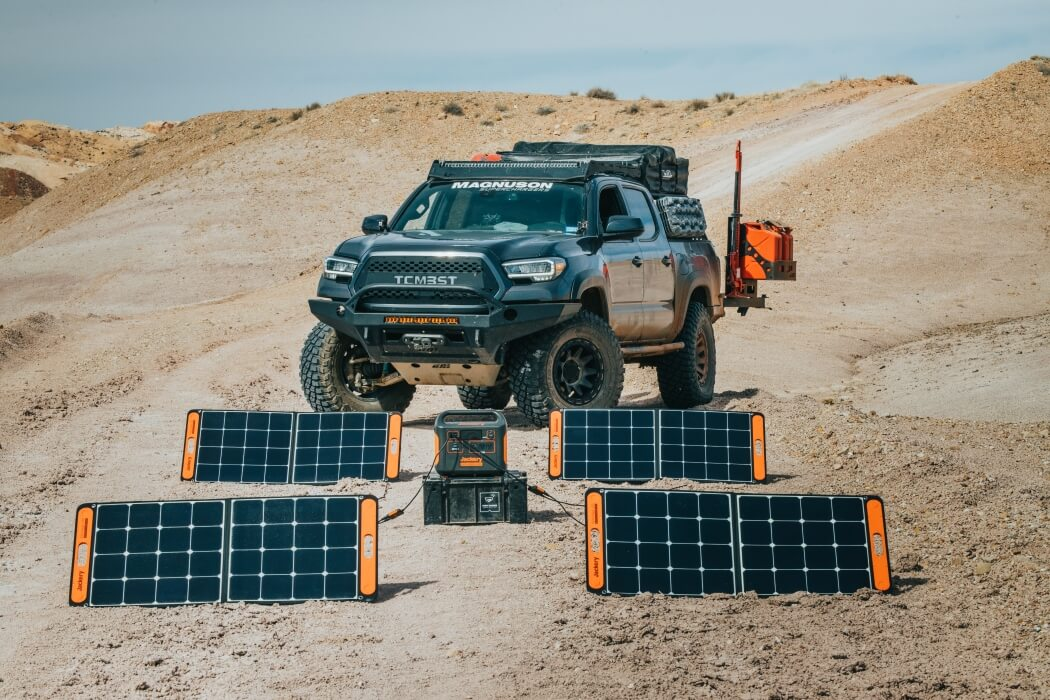You can calculate the working time using the below-mentioned formula.

Working Time = Capacity Wh × 0.85 / Operating wattage of the device = 2160 Wh  × 0.85 / 400 = 4.5 hours.

## The Importance of Watthour

Whether you plan to invest in a solar generator or want to understand your household electricity usage, learning about watthours is vital. Below we list how watthour calculation can help you.

Household Electric Energy Usage

Watthour measures the amount of energy consumed by household appliances and devices. It will help you learn how much electricity your home or outdoor appliances consume per month or year, helping you manage electricity bills efficiently.

Capacity of Power Station

Secondly, the capacity of a solar power station is measured in watt-hours. If you plan to invest in a solar generator, choosing a power station with a larger battery capacity is vital. This, in turn, ensures that the solar solution can generate enough power to meet the electrical demands for home or outdoor camping trips.

Supply and Demand Power Balance

Electrical power companies are responsible for providing a balance between the power supply and demand. Electrical energy (E) and electrical power (P) are interrelated in the below-mentioned way.

Formula: E = P × t

Where E defines the electrical energy, P is the power consumed by the appliance in time t. Power is represented in watts, while energy is measured in watthour.

The formula states energy consumption per unit of time is called power. Maintaining the power your home consumes and the supplied energy by the power station or grid is necessary to avoid fluctuations.

## Amp-Hour Vs. Watthour

Both amp-hours and watthours are units of electric charge and are interrelated.

When current (in amps) flows over a period (an hour), it is denoted as amp-hour. While watthour measures the energy, an amp-hour tells you the number of amps an appliance draws in an hour.

For example, a 100Ah battery can expel 100 amps of energy in one hour. This same 100Ah battery would expel 200 amps in two hours. To determine the battery's energy capacity, you will need to calculate both watt and amp hours.

If you have volts or amp-hours, you can easily calculate the watthours of the device and vice versa. Here is how they relate.

Formula: Watt hours = Amp hours × Volts

Or, Amp-hours = Watt hours / Volts

For instance, if you have a 12V battery for your camper or RV, and your device is rated for 100Ah, you can easily calculate the watt hours by multiplying the two.

Watt hours = 100Ah × 12V = 1200Wh.

Converting watt hours to amp-hours or vice versa is vital to understand your battery's energy capacity. With the help of calculation, you can easily calculate the number and type of batteries you require and the size of solar panels.

To help you get a quick idea, we reveal the watt and amp hours of different battery types.

 General Watt Hour Battery Capacities (with 15-hour discharge at 70 degrees F) Battery Type Lithium Lead Acid (assuming 11.8V cutoff) Voltage Capacity 12 V 24 V 12 V 24 V 50Ah 650 Wh 1300 Wh 350 Wh 700 Wh 100Ah 1300 Wh 2600 Wh 700 Wh 1400 Wh 200Ah 2600 Wh 5200 Wh 1400 Wh 2800 Wh 500Ah 6500 Wh 13000 Wh 3500 Wh 7000 Wh

## Capacities of Jackery Power Stations

Investing in the right-sized solar power station is ideal for powering appliances during power outages or outdoor adventures. Jackery Portable Power Stations are designed to charge most home appliances efficiently for long hours.

The rechargeable battery-powered generator is safe and emits no fumes. It is equipped with multiple output ports like AC outlets, USB charging ports, and a DC carport, so they can keep all your gear charged. The best part about Jackery Portable Power Stations is the Battery Management System technology that ensures a safe electricity supply to all the appliances.

Below we list the different capacities of Jackery Explorer Portable Power Stations you can choose from.

Jackery Explorer 1000 Pro Portable Power Station

With a battery capacity of 1002Wh, Jackery Explorer 1000 Pro Portable Power Station can charge 93% of most home appliances. Using four Jackery SolarSaga 200 watts solar panels, you can charge Jackery Explorer 1000 Pro in only 1.8 hours.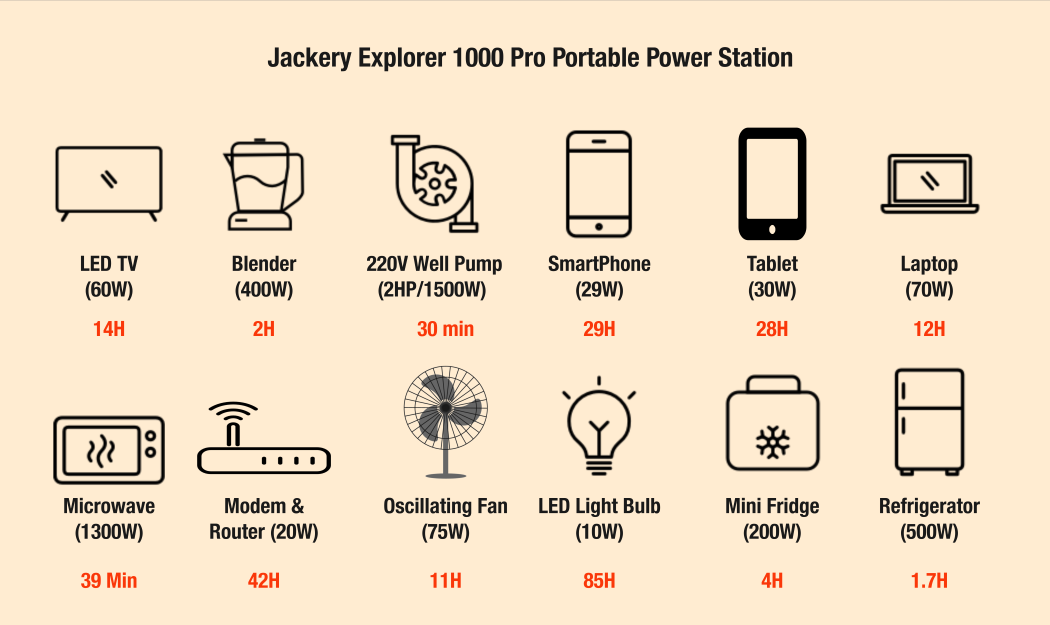Jackery Explorer 1500 Pro Portable Power Station

Equipped with a battery capacity of 1512Wh, the Jackery Explorer 1500 Pro Portable Power Station can charge 95% of most home or outdoor appliances. In addition, the intelligent BMS system and 8 state-of-the-art temperature sensors make the portable power station safe to use.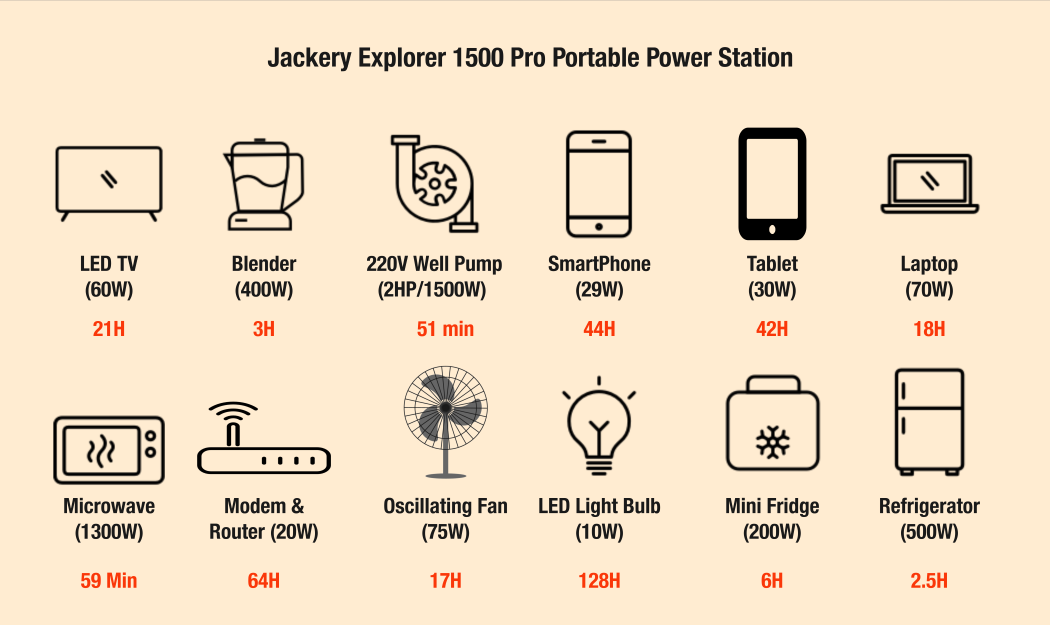Jackery Explorer 2000 Pro Portable Power Station

One of the most powerful, portable, and durable solar power stations is Jackery Explorer 2000 Pro. The first-grade lithium battery can be fully charged in under 2.5 hours using six Jackery SolarSaga 200W Solar Panels. In addition, the pass-through charging in Jackery models allows you to charge multiple devices simultaneously. If you want a solar solution that can power 96% of all home appliances, consider investing in Jackery Solar Generator 2000 Pro.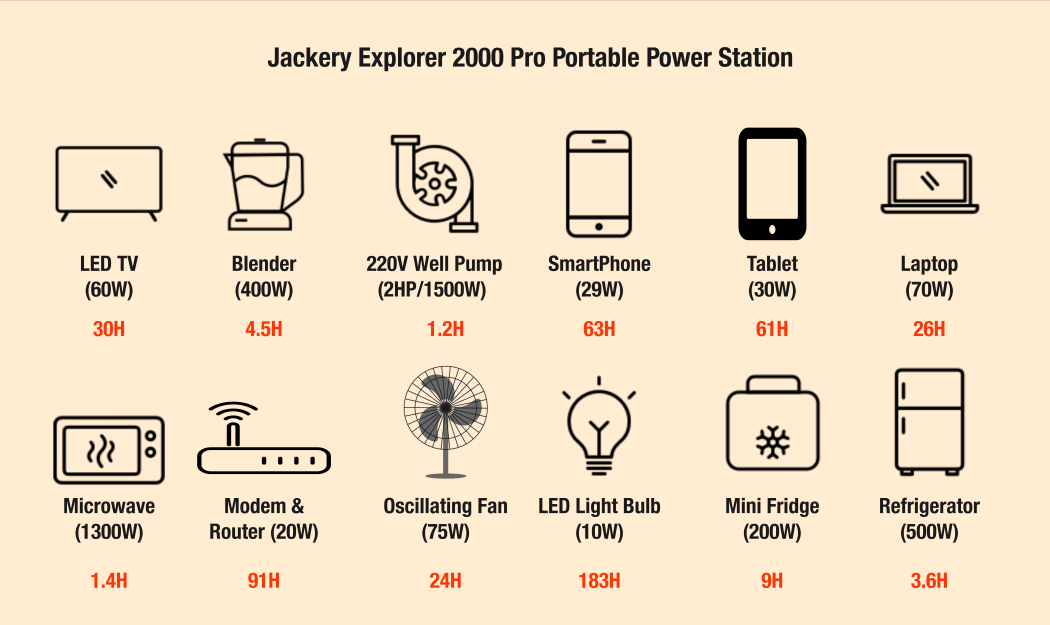Jackery Explorer Portable Power Stations are suitable for every homeowner or outdoor enthusiast looking to switch to safe and renewable solar energy.

## What Is Watthour FAQs

Now that you know "what is a watthour," here are a few frequently asked questions by the buyers of solar power stations.

How many watthours in a 100Ah lithium battery?

To calculate the watthours of a 100Ah battery, let us assume the voltage is 12V. Then, using the formula, we can calculate the estimated watthour.

Formula: Watt-hour = Amp Hour * Voltage = 100 Ah * 12V = 1200Wh.

Remember, the watthour will depend on the battery voltage. For example, if the battery voltage increases from 12V to 24V, the watthour will be 100Ah * 24V = 2400Wh.

How much is 20 watthours?

One watthour equals one watt of average power flow over an hour. Similarly, 20Wh equals 20 watts of average power flow over an hour. For example, a 20-watt bulb will consume 20Wh in one hour.

Why is it important to understand power station capacity?

Before investing in a solar power battery, it's worth noting how much power it can supply regularly. This is generally denoted by watthour or Wh. You can calculate the energy requirements of all your appliances and analyze the time you want to run them. This will help you determine the battery capacity to charge the device for long hours.

Suppose you want a solar power station for your RV appliances that consume a total of 1500 watts. In this case, you need to purchase a generator that can produce more than the required watts to function efficiently. Jackery Solar Generator 2000 Pro is the ideal solar solution that can power all your RV appliances for long hours. The Jackery Explorer 2000 Pro Portable Power Station has a battery capacity of 2160Wh that can charge most outdoor appliances.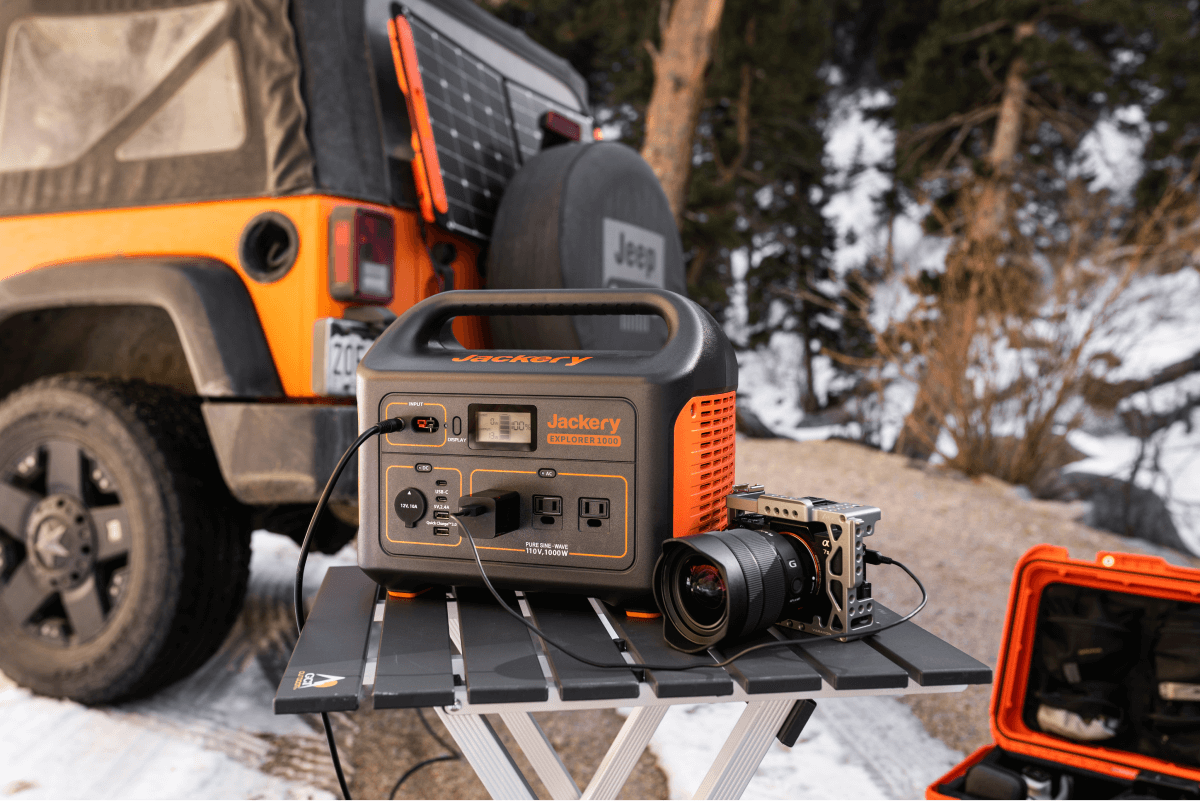## Final Thoughts

Understanding "what is a watthour" will help you know the electrical requirements of your appliances, reduce the high electricity bills, and choose a compatible solar power station. Before buying any solar solution, make sure you effectively compare the battery energy capacities.

For instance, if you want to power high-powering appliances, it's better to invest in a large-capacity portable power station. You can select Jackery Explorer 2000 Pro Portable Power Station with a battery capacity of 2160Wh to charge most small and large appliances.

On the other hand, consider investing in Jackery Explorer 2000 Pro Portable Power Station to charge most home and outdoor appliances efficiently.